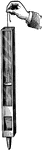Organ Pipe

"Procure an open organ-pipe, at least one side of which is made of glass. While the pipe is emitting…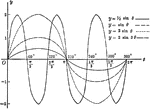Sine Curves y= sin Ǝ, y= 1/2 sin Ǝ, y=2 sin Ǝ, y= 2 sin 3Ǝ

Sine curves of varying frequency and amplitude plotted from 0 to 2 pi. Graph of y= sin θ, y= 1/2…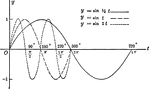Sine Curves y= sin t, y= r sin1/2t, y= r sin 2t

Sine curves of varying frequency plotted from 0 to 2 pi. Graph of y= sin t, y= r sin1/2t, y=r sin 2t.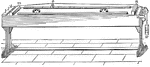Sonometer

A sonometer is an apparatus by which the transverse vibrations of strings can be studied. It is also…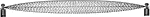Sound Wave, fundamental frequency

"Bow or pluck the string of a sonometer near its end, thus setting it in vibration as a hole. The string…